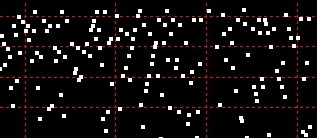exploring the undesigned
intelligence of the numberversehome   Q Factor Tessellation

First published June 2, 2008

What is a semiprime?
A natural number that is the product of two prime numbers. *

*I originally observed Q factors to be proximate to the pair of perfect squares whose difference equals N. But, of course, if a GCD exists for N and a perfect square, it also exists for N and the root of that perfect square.

Therefore, it made sense to look systematically at all numbers (odd and even) less than N to see how often and how consistently the GCD equality holds.

Try it yourself: Generate semiprimes, their Q factors, and the charts shown on this page in Excel. Download the interactive worksheet.

Graphing Q reveals an unexpected geometric thumbprint - a kind
of composite DNA - for every semiprime with distinct factors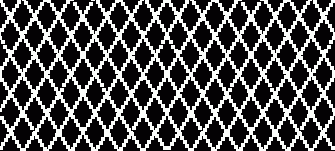Explore the phenomenon of Q Factor Tessellation with Excel Download now

Definition of Q (as a subset of N*)

For every composite N there exists a class of numbers less than N that share with N one of its factors as a GCD. In fact, we could call these numbers cofactors if that term was not already taken. (*This supersedes my original definition. )

It turns out that for what I term a symmetrical semiprime (one in which the two prime factors are distinct but are within an order of magnitude of each other), the number of Qs is about double the square root of N - sqrt(N)*2 - in other words, a lot...! (The reason for this is obvious if you read on.)

When something occurs with a frequency twice that of a square root it becomes a very attractive candidate if your hunting factors. However, for now I'm going to consider just the geometric distribution of Q for what it is: a revelation in tessellation.

So, in fact, a Q factor is any multiple of a prime factor less than N. The number of Qs for a given N must be equal to the sum of N's prime factors. Q factor tessellation occurs because, in the case of a semiprime, the cofactors of one prime factor intersect at even intervals with the cofactors of the other prime factor.

The distribution of Q exhibits a multisymmetric geometric distribution when the Qs of any non-perfect-square semiprime are graphed. For semiprimes, the patterns are remarkable: an implicate order within every N that has common elements but is yet unique for each N. Semiprimes that are perfect squares - with a prime or nonprime factor - and composites with more than two prime factors do not demonstrate Q factor tessellation.

The numbers do not look that interesting until you graph them.

 Q Q interval PS Offset GCD (Factor) 233 92 18 139 278 138 1 233 417 46 207 233 466 139 154 139 556 96 82 139 695 93 300 139 699 4 23 233 834 42 124 233 932 88 197 139 973 134 167 233 1112 139 128 139 1165 139 97 139 1251 100 128 139 1390 1 129 233 1398 8 29 233

These are the first 15 Qs of 32387 (139 * 233), showing Q intervals and perfect square
offsets (see below), and the alternating factors revealed by the GCD for each Q with N.

However, when you graph these numbers, they spring to life with brilliant precision. I've identified three distinctive types of patterns:

Q Interval Distribution - By sorting the intervals between each Q according to the size of Q (in ascending or descending order).This produces the most characteristic and consistent pattern: a diamond lattice with one row or multiple rows. The vertices of the diamonds represent areas where Qs are concentrated (where the intervals are least). The horizontal top line represents the smaller of the semiprime's factors.

Q Distribution - By sorting Q according to the size of the intervals between each Q (in ascending or descending order).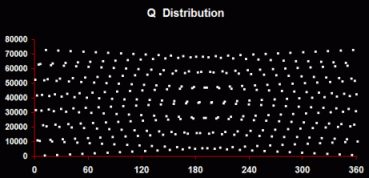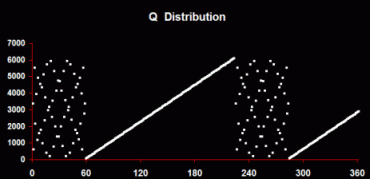This produces the widest range of patterns, from complex organized distributions, to completely linear progressions, to graphs that combine both elements.

Perfect Square Offset Distribution - The third kind of pattern - strictly, this is not tessellation - is created by looking at the differences between each Q and its immediately preceding perfect square.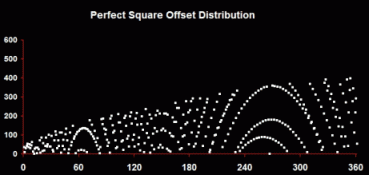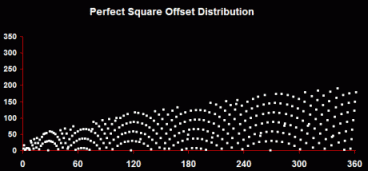These distributions produce the kind of intriguing patterns shown here - repeating curved symmetries with sprays and arches, partial or complete, as Q grows.

Don't forget that these patterns are only apparent when multiple Q factors have been found - that is, the number must be factored first - and many times. Q factors provide the means of factoring and the means of understanding an undiscovered architecture of factors.

Interim Conclusion
Can Q factors be used to factor a large number? Yes. Instead of searching for two tiny points in the square root of a semiprime, you have sqrt(N)*2 opportunities to find those two numbers in the constellation of the whole number. Does that make for better factoring odds? Yes, because we know that the distribution has certain geometric properties and that bunching occurs in the distribution at regular intervals. We know that using the birthday paradox can yield a factor by stumbling upon a cofactor surprisingly quickly. In fact, this is exactly what Pollard's rho factoring algorithm does, demonstrating why that algorithm can be so effective but unpredictable too.The only problem with this is that calculating GCDs requires many more operations than a simple divisibility test for an integer less than the square root.

Download the Excel Interactive Worksheet

Download QF1.zip (13KB)

Designed for Excel 2003. (Does NOT work with Excel 2007.)

Excel macro security should be set to Medium (Tools>Macro>Security). This gives you the choice of starting or preventing code execution when opening the workbook.

Please do NOT redistribute without the express permission of the author.

© 2008 Michael M. Ross

© 2008 Michael M. Ross